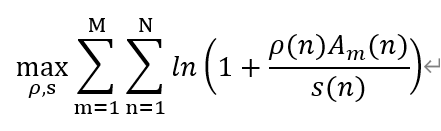# Ln(1+x/y) {convex} .* {convex}As we can see in this formula, Am(n) is constant. rho(n) and s(n) are variables,they are vectors of (1,N).This is a convex problem,but I can’t express it correctly in CVX syntax.

In my code,I express it as follows:

 R(m,n)=inv_pos(rho(n)*A(m,n))*rel_entr(rho(n)*A(m,n),s(m,n));


But there are some grammatical errors:

How can I solve this problem? Thank you!

\ln(1 + x/y) is not concave; at x=1 this is a log-sum-inv which is convex.

Oh,I got it! maybe I can solve it by converting this expression.It can be converted to

log(s(n)+rho(n)*A(m,n))-log(s(n))


the first half of this expression is concave. the second half of this expression we can use Taylor expansion to find its upper bound.
what do you think of this solution? thank you much ,Sir!

You can try whatever you want. But rather than ad hoc Taylor Series or Successive Convex Approximation /Difference of Convex (Concave), you may be better offer using a non-convex solver, for instance, under YALMIP.